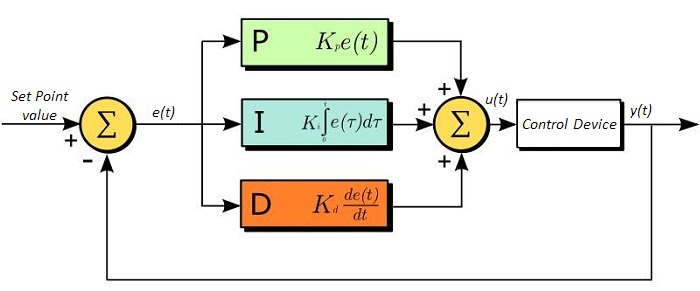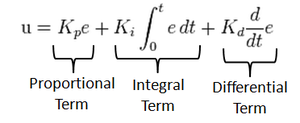## # Why PID over ‘if-else’ coding?

The Idea was to create a robot which could figure out which path he should go himself regardless of the lighting conditions. Since the average “if-else” coded robots face critical situations when there is a change of light.

The use of PID not only prevents that , but also makes the robot much more stable and reliable .The concept of PID is pretty straight forward , Instead of depending on the threshold value,PID line follower robot creates the value himself on the go. So regardless of the situation the robot can run anywhere any time , without calibrating it again and again .That’s the beauty of PID. A threshold value is a value , defined by users by calculating the value given by the IR sensors.

## # What is PID control then...

The PID control scheme is named after its three correctingterms, whose sum constitutes the manipulated variable (MV).The proportional, integral, and derivative terms are summed tocalculate the output of the PID controller.# # P Line Following Algorithm

``````void setup()
{

}
void loop()
{
int kp = 10;
int stable = 0;
int a = analog(0);
int b = analog(1);
int c = analog(3);
int d = analog(4);
int current = 2*a+b-c-2*d;

int error = stable - current;

int speed = (int) (kp * error);

lcd("%d %d %d %d %d %d",a,b,c,d,error,speed);

int  left = 100-speed;
int right = 100+speed;

if (left > 100 || left < 0 ){
left = left/100;
}
if (right > 100 || right < 0 ){
right = right/100;
}

motor(1 , left);
motor(2 , right);

}
``````
1
2
3
4
5
6
7
8
9
10
11
12
13
14
15
16
17
18
19
20
21
22
23
24
25
26
27
28
29
30
31
32
33
34
35
36
37
38
39